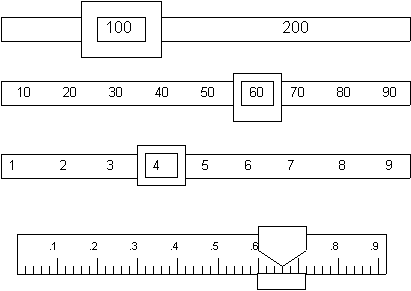### MASS, VOLUME AND DENSITY OF THREE DIFFERENT SUBSTANCES

Record the volume indicated on each of the above graduated cylinders.  Include ONE uncertain digit.  Note that the scale is different for each cylinder.What is the mass reading on the balance below?  Include ONE uncertain digit.      _________________  g.INTRODUCTION:

During this activity you will use a a graduated cylindr to measure the volume of several irregular objects by the "Displacement of Water Method"; and use a balance to obtain their respective masses.

You will then calculate the densities of these objects using the information obtained above; and use density tables to identify the substance of what these objects are made of.

PURPOSE:

Calculating mass and volume (for both regular and irregular solids) of 9 samples of three different substances; ________________, ________________, and ________________ and analyzing the density curves for each substance.

HYPOTHESIS:

Make a general prediction of what the outcome of the lab will be.

APPARATUS:

pan balance                                                           stand                                                      pencil, eraser, calculator, ruler

1 large overflow can                                            paper clip                                               2 ring clamps

graph paper                                                           1 small overflow can                            wire gauze

two 400 mL beakers

water bottle

three different sizes (small, medium, large) of 3 different substances – one of them consisting of regular shapes

METHOD:

1.     Find the mass of each object and record these values in the table.

2.     Find the volume of each of the regular-shaped objects using the method for regular solids, and record your results.

3.     Find the volume of each of the irregular-shaped objects using the method for irregular solids, and record your results.

4.     Plot a mass (g) versus volume (cm3) graph for each substance; on the same graph.  Remember which is the dependent, and which is the independent variable!!

5.     Draw the line of best fit for each substance, using different colours to represent each of them.

6.     Provide a legend for your graph.

OBSERVATIONS:

Part A:         Record your results in three tables like the following.  Remember to give each table a title!

 REGULAR SOLIDS (substance  #1) mass (g) length  (cm) width (cm) height (cm) volume (cm3) small medium large

 IRREGULAR SOLIDS (Substance #2) mass (g) volume displacement (mL) volume (cm3) small medium large IRREGULAR SOLIDS (Substance #3) mass (g) volume (mL) volume (cm3) small medium large

Part B:         Include your graph in this section.

DISCUSSION:

Part A:         Include all volume calculations made in this section (e.g. V = l x w x h).

Include all the density calculations made in this section (e.g. D = M/V)

Part B:         Answer the following questions:

1.     Which technique for measuring volume did you find to be more accurate?  Why?

2.     Explain why both mass/volume lines on the graph pass through the origin (0,0).

3.     Calculate the AVERAGE density of each substance using all three samples of each substance.

4.     From your graph determine which substance has a greater density? How did you come to this result?  Where would the curve for lead be found on your graph?  (Hint:  use p. 36 of textbook)

5.     From your graph, determine the volume of a 5 g sample of each substance?

6.     Describe some possible sources of error in any one of the techniques used in the experiment.

7.     In determining whether the three substances are made of the same material, which value - mass, volume, or density - is the only value that can be used?  Explain.

CONCLUSION:

Write a general summary of all your lab results here.
Restate the hypothesis and explain whether or not it was supported.
Were there any problems/errors in the experimentation, or in the calculation of the results?
What corrections should be made to avoid such problems?